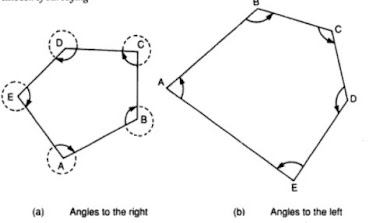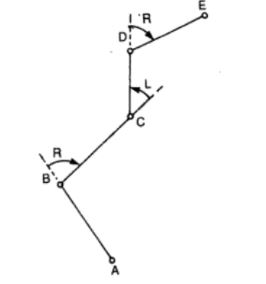# Horizontal Angles in Surveying - Deflection Angles

In surveying in lines are directions are specified using bearings and azimuths. Angles and bearings  are used to locate the points and the orientation of the line.

Note: Angles can be either horizontal or vertical. It is based on the plane in which angles are measured. A horizontal angle is measured in horizontal plane and a vertical angle is measured in vertical plane.

Horizontal angles are the basic measurements performed to determine the bearings and azimuths.

### How Are Bearings or Azimuths Measured in Surveying?

The bearings and azimuths are horizontal angles that are measured in the field using a compass, theodolite or a sextant. The angles can also be measured indirectly using chain or tape, by solving some simple geometrical figures.

### Measurement of Angles in Surveying

An angle is measured by three components:

1. Reference line
2. Direction of turning
3. Magnitude of the angular distance
These three components helps to determine the bearings and azimuths.

### Horizontal Angles in Surveying

Horizontal angles measured in surveying are of three times:
1. Interior or exterior angles
2. Angles measured to the right or left
3. Deflection angles

#### 1. Interior Angles or Exterior Angles in Surveying

Interior angles are located on the inside of a closed polygon while exterior angles are located outside a closed polygon. Exterior angles are explements of interior angles.

In surveying, it will be adequate to measure any one of them. The measurement of both the angles helps to double-check the work, because the sum of the interior and exterior angles at any station must be equal to 360 degree.Interior Angles are angles a,b and c. Exterior angle is d

#### 2. Angles Measured to the Right & Left

The interior angles measured to the right are clockwise angles. In site, the stations are designated by letter symbols and the angles are measured from the rear to forwards direction as shown in figure below.Clockwise and Anti Clockwise Angles Measured

Now, the angles are measured clockwise or anti-close wise based on how the traverse goes. If the traverse polygon moves in clockwise direction, the angles are measured in clockwise. If the traversing is moved in anti-clockwise direction, then the angle is measured in anti-clockwise direction.

3. Deflection Angles

Declination angles are angles between a line and the prolongation of the preceding line as shown in the figure below. They are measured right or left based on whether the particular line lies to the right or left of the prolongation of the preceding line.

Deflection angles have values between 0 to 180 degree and not employed for values greater than 90 degrees. The algebraic sum of the deflection angles in any closed polygon is 360 degrees. In the figure below the deflection angle measured to right is R and measured left (anti-clockwise direction) is given as L.Deflection Angles